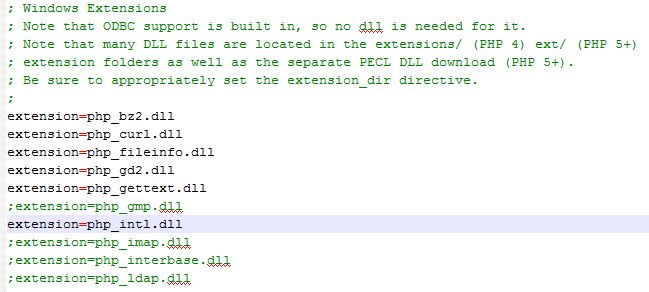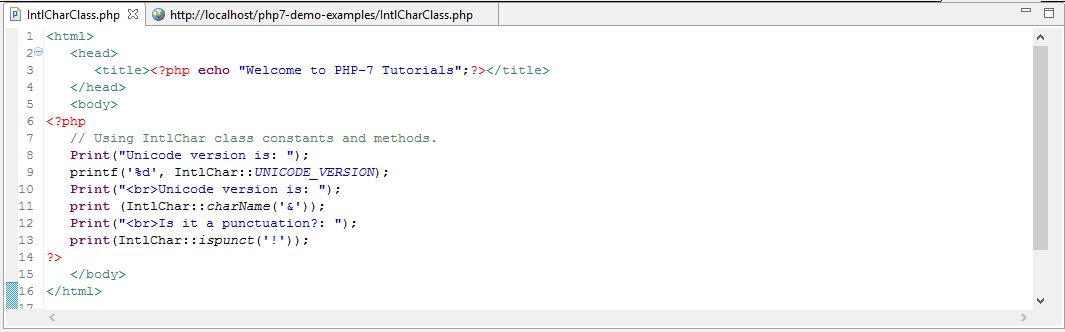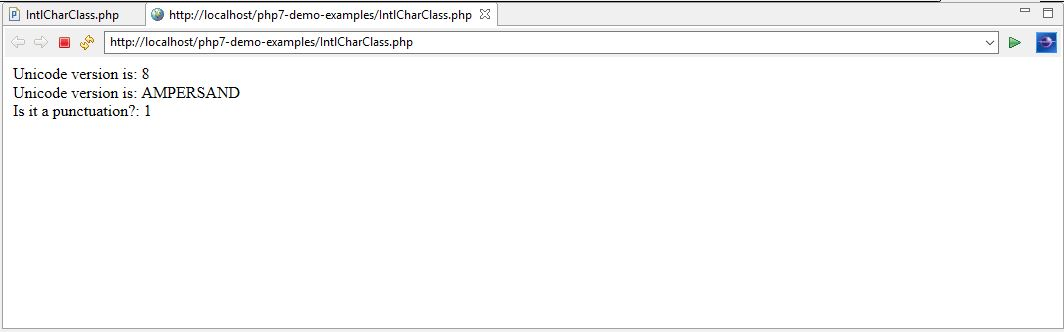# Learn How to Work with IntlChar class in PHP7

0
3908In the last chapter, we discussed about new special methods known as ‘closure call’ and ‘filtered unserialize’ with examples. In this chapter, we are going to discuss about IntlChar class that is added to PHP 7.

IntlChar class
IntlChar is a class that has been added to PHP 7. This class exposes additional ICU functionality. It has a number of constants and utility methods that can be used to access information and manipulate Unicode characters. These constants and methods resemble the names and behavior used by the underlying ICU library.

Setting up IntlChar Class
In order to use IntlChar class, we need to first install Intlextension to PHP 7. These are the following steps:

• We’ve learnt how to install and set up XAMPP for the Apache web server and PHP 7 parser on the C drive. This was covered in chapter 1 of this tutorial.
• Open the ‘C: \xampp\php\php.ini’ file in a text editor.
• From this file, remove the semicolon (;) from ‘extension=php_intl.dll’ as shown below and save the file.
• Restart the XAMPP web server.Intlextension has been installed successfully and the IntlChar class is ready to use.

Table of Content for constants present in IntlChar class

 S No Constants present in IntlChar class Implementation in PHP 7 Program 1. const string UNICODE_VERSION = 6.3 ; IntlChar::UNICODE_VERSION 2. const integer CODEPOINT_MIN = 0 ; IntlChar::CODEPOINT_MIN 3. const integer CODEPOINT_MAX = 1114111 ; IntlChar::CODEPOINT_MAX 4. const integer PROPERTY_ALPHABETIC = 0 ; IntlChar::PROPERTY_ALPHABETIC 5. const integer PROPERTY_BINARY_START = 0 ; IntlChar::PROPERTY_BINARY_START 6. const integer PROPERTY_ASCII_HEX_DIGIT = 1 ; IntlChar::PROPERTY_ASCII_HEX_DIGIT 7. const integer PROPERTY_BIDI_CONTROL = 2 ; IntlChar::PROPERTY_BIDI_CONTROL 8. const integer PROPERTY_BIDI_MIRRORED = 3 ; IntlChar::PROPERTY_BIDI_MIRRORED 9. const integer PROPERTY_DASH = 4 ; IntlChar::PROPERTY_DASH 10. const integer PROPERTY_DEFAULT_IGNORABLE_CODE_POINT = 5 ; IntlChar:: PROPERTY_DEFAULT_IGNORABLE_CODE_POINT 11. const integer PROPERTY_DEPRECATED = 6 ; IntlChar::PROPERTY_DEPRECATED 12. const integer PROPERTY_DIACRITIC = 7 ; IntlChar::PROPERTY_DIACRITIC 13. const integer PROPERTY_EXTENDER = 8 ; IntlChar::PROPERTY_EXTENDER 14. const integer PROPERTY_FULL_COMPOSITION_EXCLUSION = 9 ; IntlChar:: PROPERTY_FULL_COMPOSITION_EXCLUSION 15. const integer PROPERTY_GRAPHEME_BASE = 10 ; IntlChar::PROPERTY_GRAPHEME_BASE 16. const integer PROPERTY_GRAPHEME_EXTEND = 11 ; IntlChar::PROPERTY_GRAPHEME_EXTEND 17. const integer PROPERTY_GRAPHEME_LINK = 12 ; IntlChar::PROPERTY_GRAPHEME_LINK 18. const integer PROPERTY_HEX_DIGIT = 13 ; IntlChar::PROPERTY_HEX_DIGIT 19. const integer PROPERTY_HYPHEN = 14 ; IntlChar::PROPERTY_HYPHEN 20. const integer PROPERTY_ID_CONTINUE = 15 ; IntlChar::PROPERTY_ID_CONTINUE

Like these constants, there are around 600 constants present in IntlChar class that could be directly implemented in any PHP 7 program after enabling its extension in php.ini file.

Table of Content for utility methods present in IntlChar class

 S No. Constants present in IntlChar class Implementation in PHP 7 Program Description 1 public static array charAge ( mixed \$codepoint ) IntlChar::charAge This method gets the “age” of the code point 2 public static int charDigitValue ( mixed \$codepoint ) IntlChar::charDigitValue This method gets the decimal digit value of a decimal digit character 3 public static int charDirection ( mixed \$codepoint ) IntlChar::charDirection This method gets bidirectional category value for a code point 4 public static int charFromName ( string \$characterName [, int \$nameChoice = IntlChar::UNICODE_CHAR_NAME ] ) IntlChar::charFromName This method finds Unicode character by name and return its code point value 5 public static mixed charMirror ( mixed \$codepoint ) IntlChar::charMirror This method gets the “mirror-image” character for a code point 6 public static string charName ( mixed \$codepoint [, int \$nameChoice = IntlChar::UNICODE_CHAR_NAME ] ) IntlChar::charName This method retrieves the name of a Unicode character 7 public static int charType ( mixed \$codepoint ) IntlChar::charType This method gets the general category value for a code point 8 public static string chr ( mixed \$codepoint ) IntlChar::chr This method returns Unicode character by code point value 9 public static int digit ( string \$codepoint [, int \$radix = 10 ] ) IntlChar::digit This method gets the decimal digit value of a code point for a given radix 10 public static void enumCharNames ( mixed \$start , mixed \$limit , callable \$callback [, int \$nameChoice = IntlChar::UNICODE_CHAR_NAME ] ) IntlChar::enumCharNames This method enumerates all assigned Unicode characters within a range 11 public static void enumCharTypes ( callable \$callback ) IntlChar::enumCharTypes This method enumerates all code points with their Unicode general categories 12 public static mixed foldCase ( mixed \$codepoint [, int \$options = IntlChar::FOLD_CASE_DEFAULT ] ) IntlChar::foldCase This method performs case folding on a code point 13 public static int forDigit ( int \$digit [, int \$radix = 10 ] ) IntlChar::forDigit This method gets character representation for a given digit and radix 14 public static mixed getBidiPairedBracket ( mixed \$codepoint ) IntlChar::getBidiPairedBracket This method gets the paired bracket character for a code point 15 public static int getBlockCode ( mixed \$codepoint ) IntlChar::getBlockCode This method gets the Unicode allocation block containing a code point 16 public static int getCombiningClass ( mixed \$codepoint ) IntlChar::getCombiningClass This method gets the combining class of a code point 17 public static string getFC_NFKC_Closure ( mixed \$codepoint ) IntlChar::getFC_NFKC_Closure This method gets the FC_NFKC_Closure property for a code point 18 public static int getIntPropertyMaxValue ( int \$property ) IntlChar::getIntPropertyMaxValue This method gets the max value for a Unicode property 19 public static int getIntPropertyMinValue ( int \$property ) IntlChar::getIntPropertyMinValue This method gets the min value for a Unicode property 20 public static int getIntPropertyValue ( mixed \$codepoint , int \$property ) IntlChar::getIntPropertyValue This method gets the value for a Unicode property for a code point 21 public static float getNumericValue ( mixed \$codepoint ) IntlChar::getNumericValue This method gets the numeric value for a Unicode code point 22 public static int getPropertyEnum ( string \$alias ) IntlChar::getPropertyEnum This method gets the property constant value for a given property name 23 public static string getPropertyName ( int \$property [, int \$nameChoice = IntlChar::LONG_PROPERTY_NAME ] ) IntlChar::getPropertyName This method gets the Unicode name for a property 24 public static int getPropertyValueEnum ( int \$property , string \$name ) IntlChar::getPropertyValueEnum This method gets the property value for a given value name 25 public static string getPropertyValueName ( int \$property , int \$value [, int \$nameChoice = IntlChar::LONG_PROPERTY_NAME ] ) IntlChar::getPropertyValueName This method gets the Unicode name for a property value 26 public static array getUnicodeVersion ( void ) IntlChar::getUnicodeVersion This method gets the Unicode version 27 public static bool hasBinaryProperty ( mixed \$codepoint , int \$property ) IntlChar::hasBinaryProperty This method checks a binary Unicode property for a code point 28 public static bool isalnum ( mixed \$codepoint ) IntlChar::isalnum This method checks if code point is an alphanumeric character 29 public static bool isalpha ( mixed \$codepoint ) IntlChar::isalpha This method checks if the code point is a letter character 30 public static bool isbase ( mixed \$codepoint ) IntlChar::isbase This method checks if the code point is a base character 31 public static bool isblank ( mixed \$codepoint ) IntlChar::isblank This method checks if the code point is a “blank” or “horizontal space” character 32 public static bool iscntrl ( mixed \$codepoint ) IntlChar::iscntrl This method checks if the code point is a control character 33 public static bool isdefined ( mixed \$codepoint ) IntlChar::isdefined This method checks whether the code point is defined 34 public static bool isdigit ( mixed \$codepoint ) IntlChar::isdigit This method checks if the code point is a digit character 35 public static bool isgraph ( mixed \$codepoint ) IntlChar::isgraph This method checks if the code point is a graphic character 36 public static bool isIDIgnorable ( mixed \$codepoint ) IntlChar::isIDIgnorable This method checks if the code point is an ignorable character 37 public static bool isIDPart ( mixed \$codepoint ) IntlChar::isIDPart This method checks if the code point is permissible in an identifier 38 public static bool isIDStart ( mixed \$codepoint ) IntlChar::isIDStart This method checks if the code point is permissible as the first character in an identifier 39 public static bool isISOControl ( mixed \$codepoint ) IntlChar::isISOControl This method checks if the code point is an ISO control code 40 public static bool isJavaIDPart ( mixed \$codepoint ) IntlChar::isJavaIDPart This method checks if the code point is permissible in a Java identifier 41 public static bool isJavaIDStart ( mixed \$codepoint ) IntlChar::isJavaIDStart This method checks if the code point is permissible as the first character in a Java identifier 42 public static bool isJavaSpaceChar ( mixed \$codepoint ) IntlChar::isJavaSpaceChar This method checks if the code point is a space character according to Java 43 public static bool islower ( mixed \$codepoint ) IntlChar::islower This method checks if the code point is a lowercase letter 44 public static bool isMirrored ( mixed \$codepoint ) IntlChar::isMirrored This method checks if the code point has the Bidi_Mirrored property 45 public static bool isprint ( mixed \$codepoint ) IntlChar::isprint This method checks if the code point is a printable character 46 public static bool ispunct ( mixed \$codepoint ) IntlChar::ispunct This method checks if the code point is punctuation character 47 public static bool isspace ( mixed \$codepoint ) IntlChar::isspace This method checks if the code point is a space character 48 public static bool istitle ( mixed \$codepoint ) IntlChar::istitle This method checks if the code point is a Titlecase letter 49 public static bool isUAlphabetic ( mixed \$codepoint ) IntlChar::isUAlphabetic This method checks if the code point has the Alphabetic Unicode property 50 public static bool isULowercase ( mixed \$codepoint ) IntlChar::isULowercase This method checks if the code point has the Lowercase Unicode property 51 public static bool isupper ( mixed \$codepoint ) IntlChar::isupper This method checks if the code point has the general category “Lu” (uppercase letter) 52 public static bool isUUppercase ( mixed \$codepoint ) IntlChar::isUUppercase This method checks if the code point has the Uppercase Unicode property 53 public static bool isUWhiteSpace ( mixed \$codepoint ) IntlChar::isUWhiteSpace This method checks if the code point has the White_Space Unicode property 54 public static bool isWhitespace ( mixed \$codepoint ) IntlChar::isWhitespace This method checks if the code point is a whitespace character according to ICU 55 public static bool isxdigit ( mixed \$codepoint ) IntlChar::isxdigit This method checks if the code point is a hexadecimal digit 56 public static int ord ( mixed \$character ) IntlChar::ord This method returns the Unicode code point value of character 57 public static mixed tolower ( mixed \$codepoint ) IntlChar::tolower This method makes the Unicode character lowercase 58 public static mixed totitle ( mixed \$codepoint ) IntlChar::totitle This method makes the Unicode character titlecase 59 public static mixed toupper ( mixed \$codepoint ) IntlChar::toupper This method makes the Unicode character uppercase

PHP 7 Code example on IntlChar class
In the example below, we are just implementing few constants and utility method of IntlChar class.Explanation of code

• By using IntlChar::UNICODE_VERSION, we are printing the version number which is 8.
• By using the utility method IntlChar::charName (‘&’), we are printing the name of the character, which is ‘AMPERSAND’ here.
• By using the utility method IntlChar::ispunct (‘!’), we are printing the Boolean value. Here, it is 1 which means true.

Output
As explained above, following is the output.